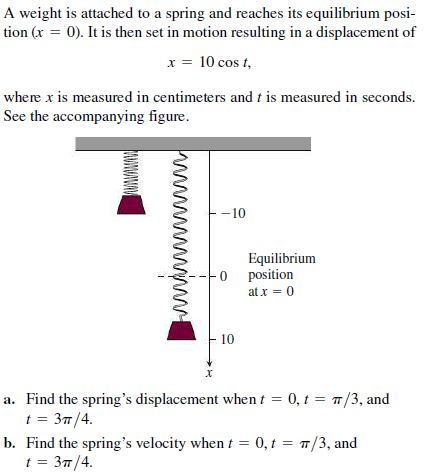# A weight is attached to a spring and reaches its equilibrium posi- tion (x = 0). It is then set in motion resulting in a displacement of x = 10 cos t, where x is measured in centimeters and t is measured in seconds. See the accompanying figure. -10 Equilibrium position at x = 0 10 a. Find the spring's displacement when t = 0, t = 7/3, and t = 37/4. b. Find the spring's velocity when t = 0, t = T/3, and t = 37/4. www

Questionhelp_outlineImage TranscriptioncloseA weight is attached to a spring and reaches its equilibrium posi- tion (x = 0). It is then set in motion resulting in a displacement of x = 10 cos t, where x is measured in centimeters and t is measured in seconds. See the accompanying figure. -10 Equilibrium position at x = 0 10 a. Find the spring's displacement when t = 0, t = 7/3, and t = 37/4. b. Find the spring's velocity when t = 0, t = T/3, and t = 37/4. www fullscreen

### Want to see this answer and more?

Experts are waiting 24/7 to provide step-by-step solutions in as fast as 30 minutes!*

*Response times may vary by subject and question complexity. Median response time is 34 minutes for paid subscribers and may be longer for promotional offers.
Tagged in
Math
Calculus

### Applications of Derivative